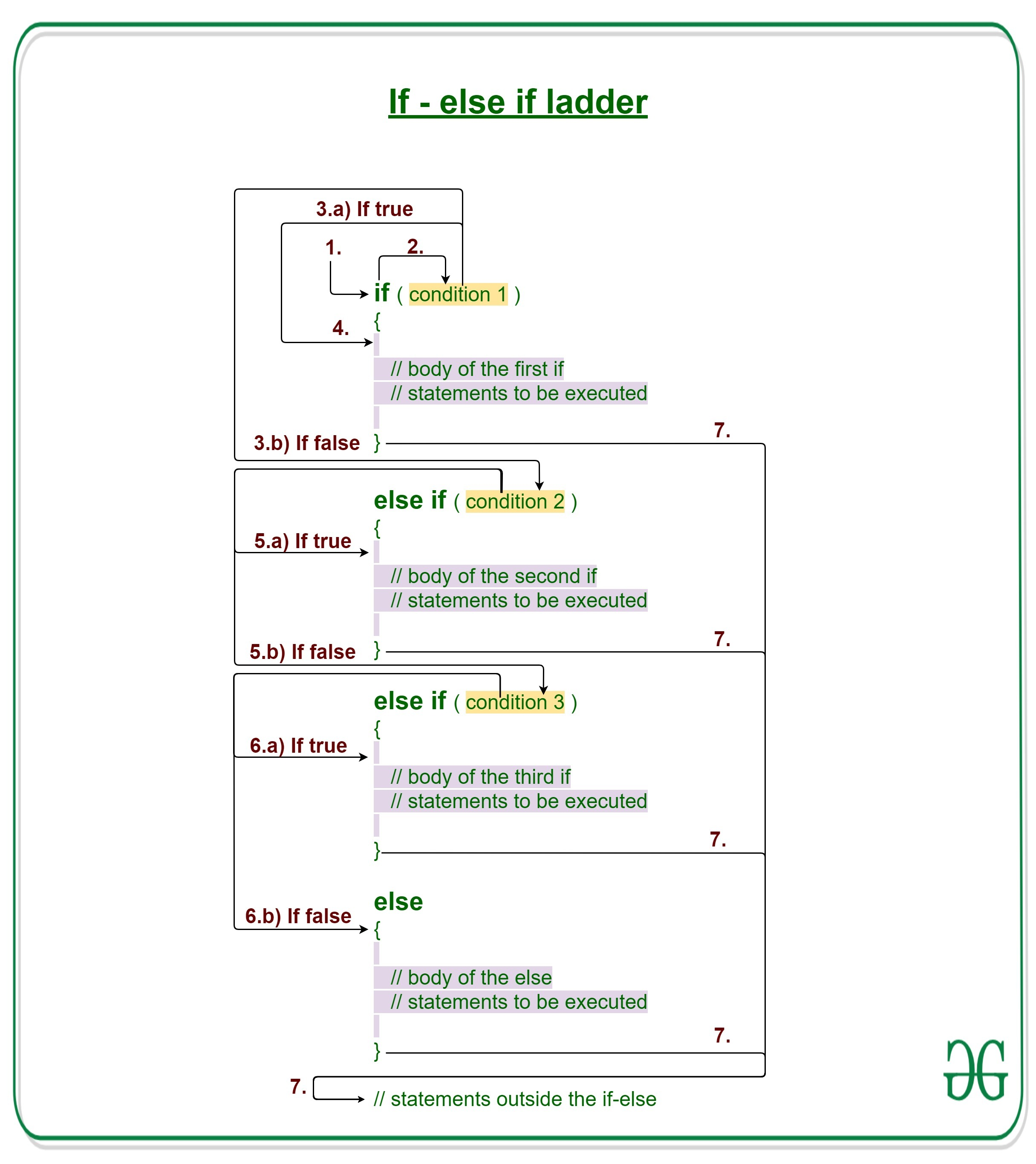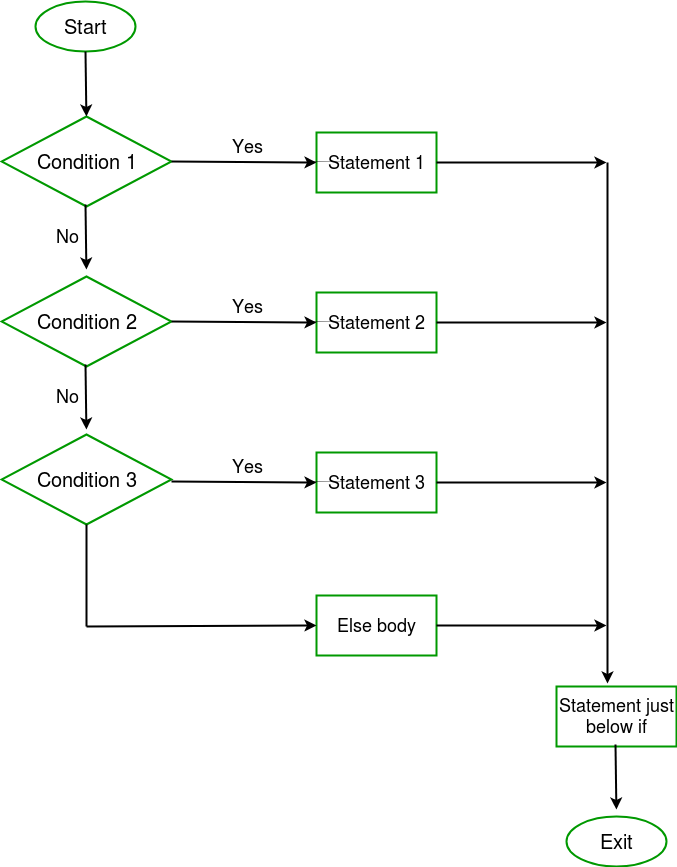# C/C++ if else if ladder with Examples

Decision Making in C/C++ helps to write decision driven statements and execute a particular set of code based on certain conditions.

In C/C++ if-else-if ladder helps user decide from among multiple options. The C/C++ if statements are executed from the top down. As soon as one of the conditions controlling the if is true, the statement associated with that if is executed, and the rest of the C else-if ladder is bypassed. If none of the conditions is true, then the final else statement will be executed.Syntax:

```if (condition)
statement 1;
else if (condition)
statement 2;
.
.
else
statement;```

1. Control falls into the if block.
2. The flow jumps to Condition 1.
3. Condition is tested.
1. If Condition yields true, goto Step 4.
2. If Condition yields false, goto Step 5.
4. The present block is executed. Goto Step 7.
5. The flow jumps to Condition 2.
1. If Condition yields true, goto step 4.
2. If Condition yields false, goto Step 6.
6. The flow jumps to Condition 3.
1. If Condition yields true, goto step 4.
2. If Condition yields false, execute else block. Goto Step 7.Example 1:

## C

 `// C program to illustrate nested-if statement ` ` `  `#include ` ` `  `int` `main() ` `{ ` `    ``int` `i = 20; ` ` `  `    ``// Check if i is 10 ` `    ``if` `(i == 10) ` `        ``printf``(``"i is 10"``); ` ` `  `    ``// Since i is not 10 ` `    ``// Check if i is 15 ` `    ``else` `if` `(i == 15) ` `        ``printf``(``"i is 15"``); ` ` `  `    ``// Since i is not 15 ` `    ``// Check if i is 20 ` `    ``else` `if` `(i == 20) ` `        ``printf``(``"i is 20"``); ` ` `  `    ``// If none of the above conditions is true ` `    ``// Then execute the else statement ` `    ``else` `        ``printf``(``"i is not present"``); ` ` `  `    ``return` `0; ` `} `

## C++

 `// C++ program to illustrate if-else-if ladder ` `#include ` `using` `namespace` `std; ` ` `  `int` `main() ` `{ ` `    ``int` `i = 20; ` ` `  `    ``// Check if i is 10 ` `    ``if` `(i == 10) ` `        ``cout << ``"i is 10"``; ` ` `  `    ``// Since i is not 10 ` `    ``// Check if i is 15 ` `    ``else` `if` `(i == 15) ` `        ``cout << ``"i is 15"``; ` ` `  `    ``// Since i is not 15 ` `    ``// Check if i is 20 ` `    ``else` `if` `(i == 20) ` `        ``cout << ``"i is 20"``; ` ` `  `    ``// If none of the above conditions is true ` `    ``// Then execute the else statement ` `    ``else` `        ``cout << ``"i is not present"``; ` ` `  `    ``return` `0; ` `} `

Output:

```i is 20
```

Dry running Example 1:

```1. Program starts.
2. i is initialized to 20.
3. condition 1 is checked. 20 == 10, yields false.
4. condition 2 is checked. 20 == 15, yields false.
5. condition 3 is checked. 20 == 20, yields true.
5.a) "i is 20" gets printed.
6. "Outside if-else-if" gets printed.
7. Program ends.```

Example 2:

## C

 `// C program to illustrate nested-if statement ` ` `  `#include ` ` `  `int` `main() ` `{ ` `    ``int` `i = 25; ` ` `  `    ``// Check if i is between 0 and 10 ` `    ``if` `(i >= 0 && i <= 10) ` `        ``printf``(``"i is between 0 and 10"``); ` ` `  `    ``// Since i is not between 0 and 10 ` `    ``// Check if i is between 11 and 15 ` `    ``else` `if` `(i >= 11 && i <= 15) ` `        ``printf``(``"i is between 11 and 15"``); ` ` `  `    ``// Since i is not between 11 and 15 ` `    ``// Check if i is between 16 and 20 ` `    ``else` `if` `(i >= 16 && i <= 20) ` `        ``printf``(``"i is between 16 and 20"``); ` ` `  `    ``// Since i is not between 0 and 20 ` `    ``// It means i is greater than 20 ` `    ``else` `        ``printf``(``"i is greater than 20"``); ` `} `

## C++

 `// C++ program to illustrate if-else-if ladder ` ` `  `#include ` `using` `namespace` `std; ` ` `  `int` `main() ` `{ ` `    ``int` `i = 25; ` ` `  `    ``// Check if i is between 0 and 10 ` `    ``if` `(i >= 0 && i <= 10) ` `        ``cout << ``"i is between 0 and 10"` `<< endl; ` ` `  `    ``// Since i is not between 0 and 10 ` `    ``// Check if i is between 11 and 15 ` `    ``else` `if` `(i >= 11 && i <= 15) ` `        ``cout << ``"i is between 11 and 15"` `<< endl; ` ` `  `    ``// Since i is not between 11 and 15 ` `    ``// Check if i is between 16 and 20 ` `    ``else` `if` `(i >= 16 && i <= 20) ` `        ``cout << ``"i is between 16 and 20"` `<< endl; ` ` `  `    ``// Since i is not between 0 and 20 ` `    ``// It means i is greater than 20 ` `    ``else` `        ``cout << ``"i is greater than 20"` `<< endl; ` `} `

Output:

```i is greater than 20
```

Related Articles:

GeeksforGeeks has prepared a complete interview preparation course with premium videos, theory, practice problems, TA support and many more features. Please refer Placement 100 for details

My Personal Notes arrow_drop_upCheck out this Author's contributed articles.

If you like GeeksforGeeks and would like to contribute, you can also write an article using contribute.geeksforgeeks.org or mail your article to contribute@geeksforgeeks.org. See your article appearing on the GeeksforGeeks main page and help other Geeks.

Please Improve this article if you find anything incorrect by clicking on the "Improve Article" button below.

Article Tags :
Practice Tags :

Be the First to upvote.

Please write to us at contribute@geeksforgeeks.org to report any issue with the above content.